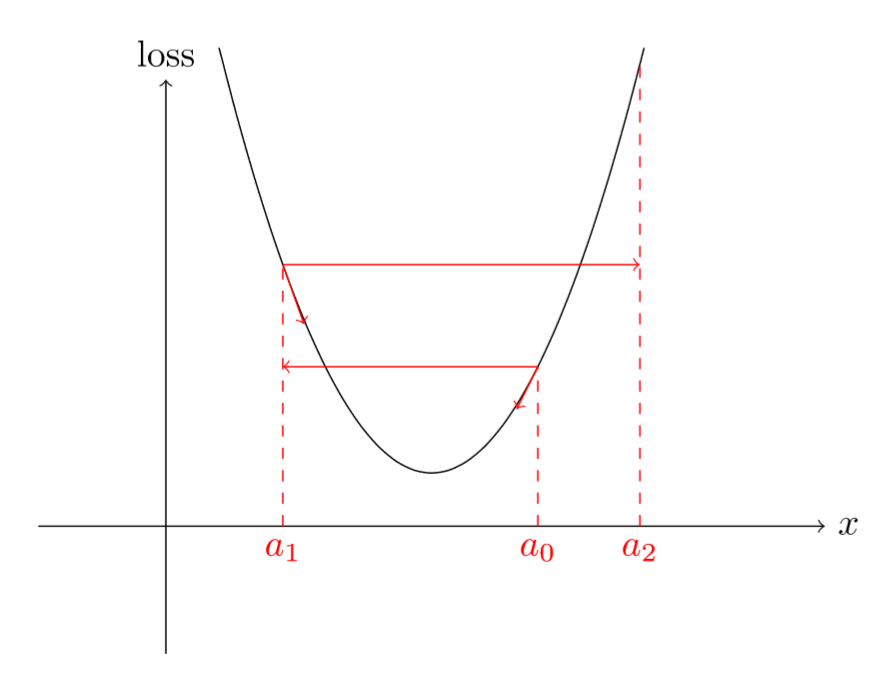learner.lr_find()
learner.sched.plot()()def find_lr(init_value = 1e-8, final_value=10., beta = 0.98):
mult = (final_value / init_value) ** (1/num)
lr = init_value
optimizer.param_groups['lr'] = lr
avg_loss = 0.
best_loss = 0.
batch_num = 0
losses = []
log_lrs = []
batch_num += 1
#As before, get the loss for this mini-batch of inputs/outputs
inputs,labels = data
inputs, labels = Variable(inputs), Variable(labels)
outputs = net(inputs)
loss = criterion(outputs, labels)
#Compute the smoothed loss
avg_loss = beta * avg_loss + (1-beta) *loss.data
smoothed_loss = avg_loss / (1 - beta**batch_num)
#Stop if the loss is exploding
if batch_num > 1 and smoothed_loss > 4 * best_loss:
return log_lrs, losses
#Record the best loss
if smoothed_loss < best_loss or batch_num==1:
best_loss = smoothed_loss
#Store the values
losses.append(smoothed_loss)
log_lrs.append(math.log10(lr))
#Do the SGD step
loss.backward()
optimizer.step()
#Update the lr for the next step
lr *= mult
optimizer.param_groups['lr'] = lr
return log_lrs, losses